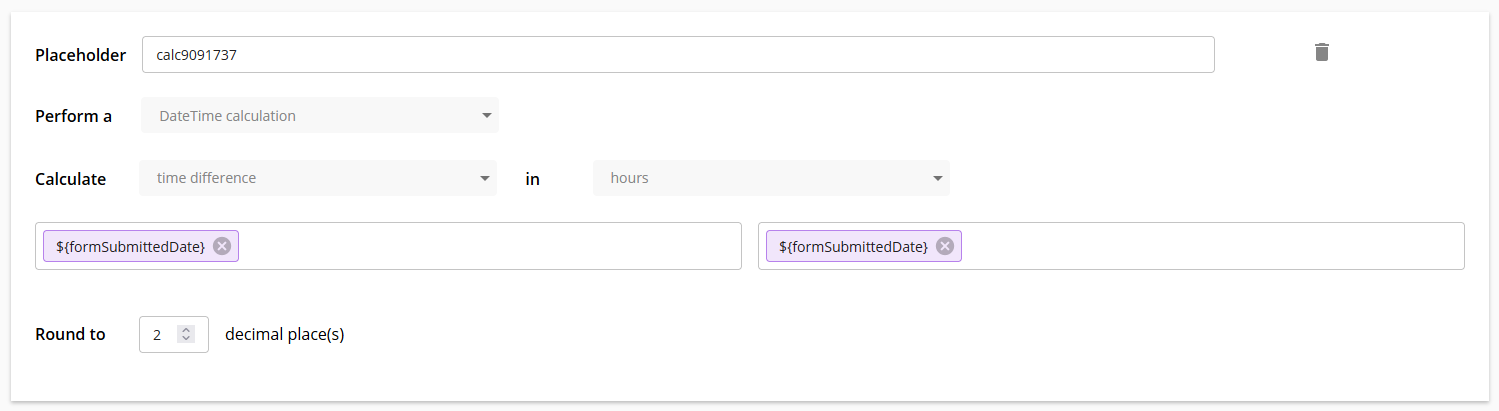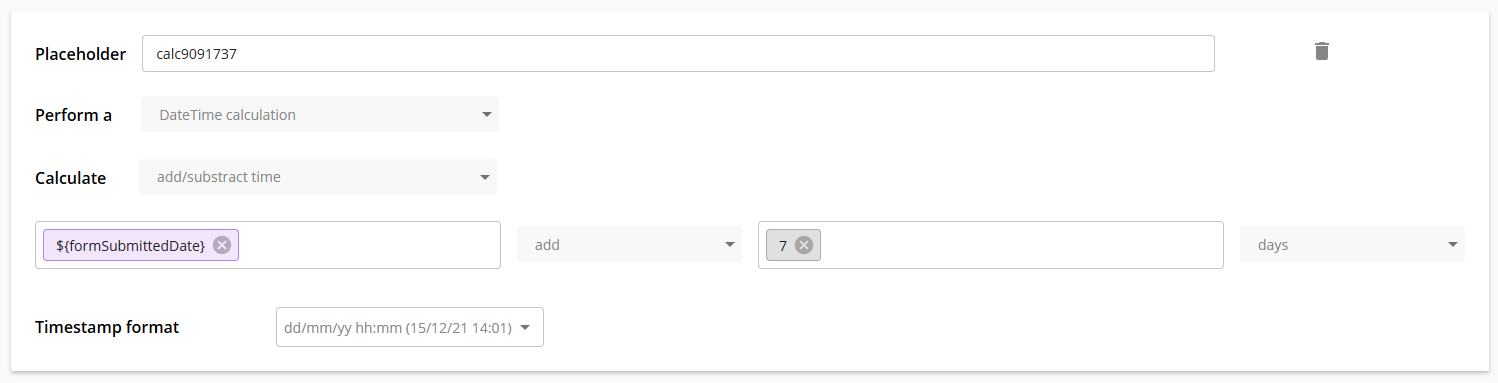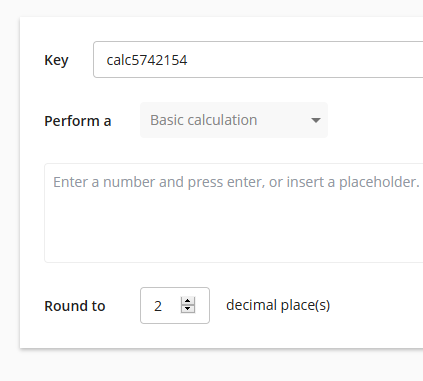# Calculator

ComponentTypeDescriptionCalculator🔀 actionperform calculations including statistical functions

The calculator component allows you to perform calculations and functions. There are two calculation modes.

### Basic calculation#

In basic calculation mode, you can use a combination of numbers, operators and placeholders to create a formula.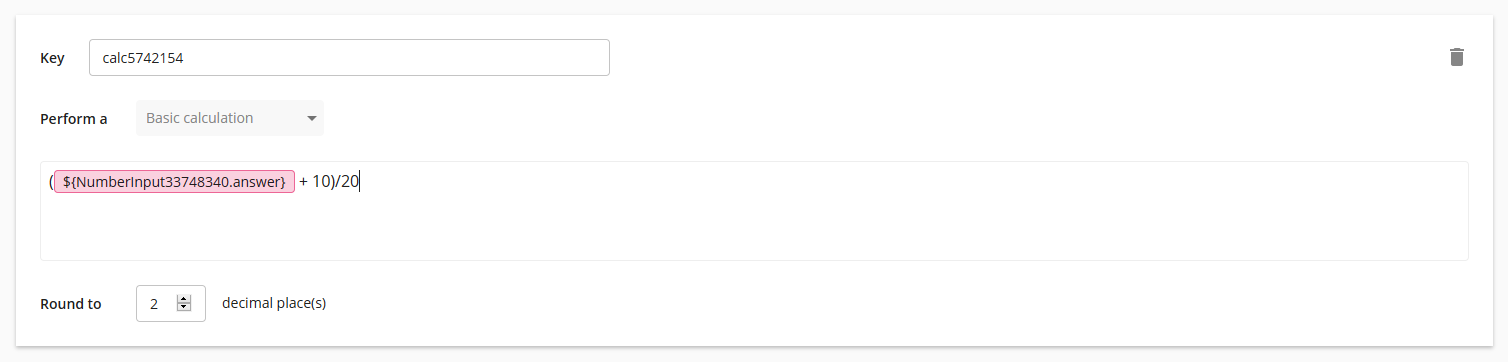The operators you can use in the basic calculation mode are:

• “+” to sum e.g. 2+2 = 4
• “-” to subtract e.g. 4-2 = 2
• “*” to multiply e.g. 22 = 4
• “/” to divide e.g. 4/2 = 2
• “^” to power e.g. 2^2 = 4
• “(“ and “)” to place brackets e.g. (2+2)/4 = 1

### Function#

In the function mode, you can select from a menu of various functions and use a combination of numbers and placeholders to perform these functions.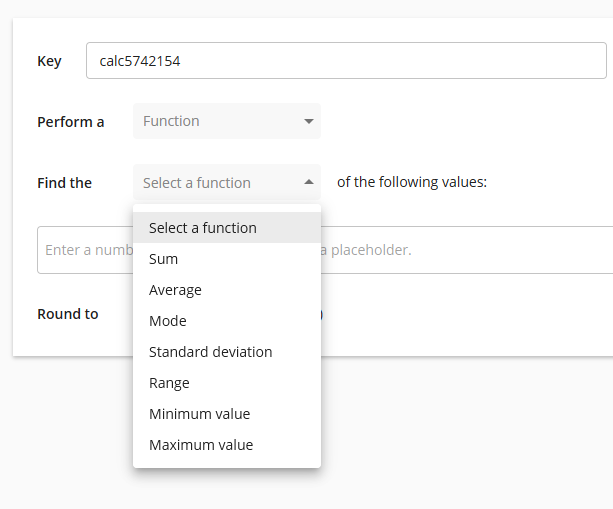The function mode works on a set of values which can be number placeholders or numbers. To manually enter numbers into the value set, type the number and press enter.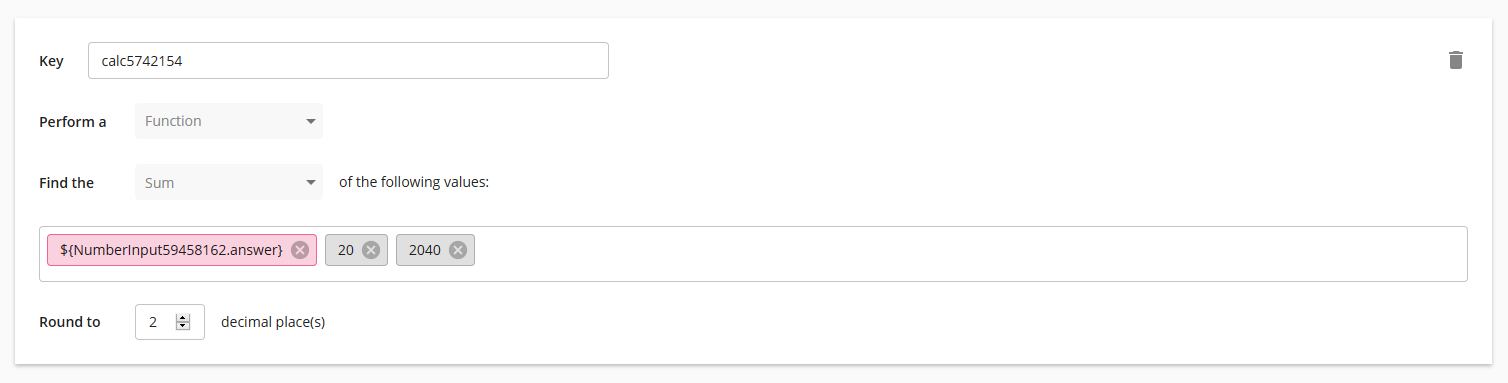The following functions can be applied to the value set:

• “Sum” adds all numbers and placeholders entered
• “Average” finds the average/mean of all numbers and placeholders entered
• “Mode” finds the value that appears most often in set of values entered
• “Standard deviation“ returns the standard deviation of the set of values entered
• “Range” finds the numerical range of the set of values entered
• “Minimum value” will return the smallest of the set of values entered
• “Maximum value” will return the largest of the set of values entered

### Datetime calculations#

You can also perform calculations on datetime values such as adding time to a datetime value, or finding the difference between two datetime values.

#### Difference between two datetime values#

This function returns the time difference between two datetime values in the selected unit e.g. difference between 12 August 2021 and 14 August 2021 in days = 2 days.